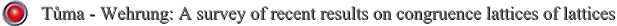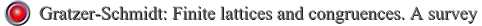Congruence lattices of lattices

 There exists a distributive algebraic lattice which is not isomorphic to the congruence lattice of any lattice. This lattice has א ω+1 compact elements. There is a distributive join semilattice S of size  אi2  that is not isomorphic to the congruence lattice of compact congruences of any lattice. There is a distributive algebraic lattice that is not isomorphic to the congruence lattice of any algebra with permutable congruences. Local separation in distributive semilattices, AU 54, 323-335

Papers:Open  problems

Problem 1. Does there exists a distributive algebraic lattice which is not isomorphic to the congruence lattice of any algebraic structure with only finitely many operations ? What lattices are congruence lattices of algebras with only finitely many operations?

Problem 2. ( Lampe)  Is there an algebra A with exactly  אi0 operations such that there is no B with only finitely many operations and with Con(B) isomorphic to Con(A) ?

Problem 3. Is every finite distributive lattice isomorphic to the congruence lattice of an isoform modular lattice ?

Problem 4. (Lampe) Which algebraic lattices are isomorphic to the congruence lattices of algebras having one element subalgebras ?